# Graphical Methods for Categorical Data

Michael Friendly, York University, 4700 Keele Street, Toronto, ON, Canada M3J 1P3
Paper presented at the SAS SUGI 17 Conference, April,1992

# Abstract

Statistical methods for categorical data, such as loglinear models and logistic regression, represent discrete analogs of the analysis of variance and regression methods for continuous response variables. However, while graphical display techniques are common adjuncts to analysis of variance and regression, methods for plotting contingency table data are not as widely used.

This paper provides a brief introduction to graphical methods that are useful for understanding the pattern of association among categorical variables. These methods can be helpful both for data exploration and for communicating results to others. The methods described include association plots for two-way tables, mosaic displays for multiway tables, correspondence analysis and effect plots for logit models.

# Introduction

Graphical methods for quantitative data are well developed. From the basic display of data in a scatterplot, to diagnostic methods for assessing assumptions and finding transformations, to the final presentation of results, graphical techniques are commonplace adjuncts to most methods of statistical analysis. In contrast, graphical methods for categorical data are still in infancy. There are not many methods, those that are available in the literature are not accessible in common statistical software, and consequently they are not widely used. This contrast between graphical methods for quantitative vs. qualitative data leads to the following observations:
• Exploratory methods: Many of the graphical methods described here make minimal assumptions about the data. Their goal is to help the viewer see the data, detect patterns, and suggest hypotheses.
• Graphic metaphor: The visual metaphor for displaying quantitative data is magnitude ~ position along an axis. Some of the methods described here (e.g., sieve diagram, mosaic display) suggest that the appropriate visual metaphor for counts of observations in discrete categories is count ~ area.
• Generalizations?: The scatterplot is a basic tool for viewing raw (quantitative) data. It generalizes readily to three or more variables in the form of the scatterplot matrix -- a matrix of pairwise scatterplots. The mosaic display is a simple graphic method for looking at cross-classified data which generalizes to more than two-way tables. Are there others?
• Presentation plots for model-based methods: Results of model-based analysis are almost invariably presented in tables of estimated frequencies, parameter estimates, log-linear model effects, and so forth. Effect displays of estimated probabilities of response or log odds provide a useful alternative.
• Practical power = Statistical power * Probability of Use: Statistical and graphical methods are of practical value to the extent that they are available and easy to use. Statistical methods for categorical data analysis have nearly reached that point. Graphical methods still have a long way to go. One aim for this paper is to show what can now be done, with some examples of how to do it.
The graphical displays shown here are implemented in SAS/IML software whose combination of matrix operations, built-in functions for contingency table analysis, and graphics provide a convenient environment for graphical display for multiway categorical data (Friendly 1991a; 1992).

# Plots for two-way frequency tables

Several schemes for representing contingency tables graphically are based on the fact that when the row and column variables are independent, the estimated expected frequencies, e sub ij, are products of the row and column totals (divided by the grand total). Then, each cell can be represented by a rectangle whose area shows the cell frequency, f sub ij, or deviation from independence.

## Sieve diagrams

Table 1 shows data on the relation between hair color and eye color among 592 subjects (students in a statistics course) collected by Snee (1974). The Pearson chi sup 2 for these data is 138.3 with 9 degrees of freedom, indicating substantial departure from independence. The question is how to understand the nature of the association between hair and eye color.
```

Table 1:   Hair-color eye-color data

Hair Color
Eye
Color     BLACK    BROWN      RED    BLOND  | Total
|
Brown        68      119       26        7  |   220
Blue         20       84       17       94  |   215
Hazel        15       54       14       10  |    93
Green         5       29       14       16  |    64
--------------------------------------------+------
Total       108      286       71      127  |   592

```

For any two-way table, the expected frequencies under independence can be represented by rectangles whose widths are proportional to the total frequency in each column, f sub +j, and whose heights are proportional to the total frequency in each row, f sub i+; the area of each rectangle is then proportional to e sub ij. Figure 1 shows the expected frequencies for the hair and eye color data.Figure 1: Expected frequencies under independence

Riedwyl and Schupbach (1983, 1994) proposed a sieve diagram (later called a parquet diagram ) based on this principle. In this display the area of each rectangle is proportional to expected frequency and observed frequency is shown by the number of squares in each rectangle. Hence, the difference between observed and expected frequency appears as the density of shading, using color to indicate whether the deviation from independence is positive or negative. (In monochrome versions, positive deviations are shown by solid lines, negative by broken lines.) The sieve diagram for hair color and eye color is shown in Figure 2.Figure 2: Sieve diagram for hair-eye data

## Association plot for two-way tables

In the sieve diagram the foreground (rectangles) shows expected frequencies; deviations from independence are shown by color and density of shading. The association plot (Cohen, 1980; Friendly, 1991a) puts deviations from independence in the foreground: the area of each box is made proportional to observed - expected frequency.

For a two-way contingency table, the signed contribution to Pearson chi sup 2 for cell i, j is d sub ij = ( f sub ij - e sub ij ) / sqrt e sub ij , so that chi sup 2 = Sigma Sigma sub ij d sub ij sup 2. In the association plot , each cell is shown by a rectangle that has (signed) height &prop d sub ij and width proportional to sqrt e sub ij. The area of each rectangle is therefore proportional to f sub ij - e sub ij. The rectangles for each row in the table are positioned relative to a baseline representing independence (d sub ij = 0) shown by a dotted line. Cells with observed > expected frequency rise above the line (and are colored black); cells that contain less than the expected frequency fall below it (and are shaded red). Figure 3 shows the association plot for the hair-eye color data.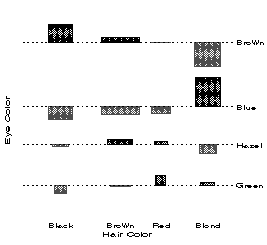Figure 3: Association plot for hair-eye data

## Four-fold display for 2 x 2 tables

For a 2 x 2 table, the departure from independence can be measured by the sample odds ratio, theta = (f sub 11 / f sub 12 ) / (f sub 21 / f sub 22 ). The four-fold display shows the frequencies in a 2 x 2 table in a way that depicts the odds ratio. In this display the frequency in each cell is shown by a quarter circle, whose radius is proportional to sqrt f sub ij, so again area is proportional to count. An association between the variables (odds ratio != 1) is shown by the tendency of diagonally opposite cells in one direction to differ in size from those in the opposite direction, and we use color and shading to show this direction. If the marginal proportions in the table differ markedly, the table may first be standardized (using iterative proportional fitting) to a table with equal margins but the same odds ratio.

Figure 4 shows aggregate data on applicants to graduate school at Berkeley for the six largest departments in 1973 classified by admission and gender. At issue is whether the data show evidence of sex bias in admission practices (Bickel et al., 1975). The figure shows the cell frequencies numerically, but margins for both sex and admission are equated in the display. For these data the sample odds ratio, Odds (Admit|Male) / (Admit|Female) is 1.84 indicating that males are almost twice as likely in this sample to be admitted. The four-fold display shows this imbalance clearly.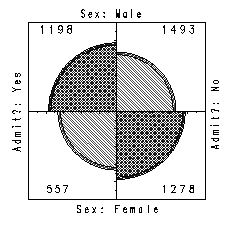Figure 4: Four-fold display for Berkeley admissions. The area of each shaded quadrant shows the frequency, standardized to equate the margins for sex and admission. Circular arcs show the limits of a 99% confidence interval for the odds ratio.

# Mosaic displays for n-way tables

The mosaic display, proposed by Hartigan & Kleiner (1981), represents the counts in a contingency table directly by tiles whose area is proportional to the cell frequency. This display generalizes readily to n-way tables and can be used to display the residuals from various log-linear models.

One form of this plot, called the condensed mosaic display , is similar to a divided bar chart. The width of each column of tiles in Figure 5 is proportional to the marginal frequency of hair colors. Again, the area of each box is proportional to the cell frequency, and complete independence is shown when the tiles in each row all have the same height.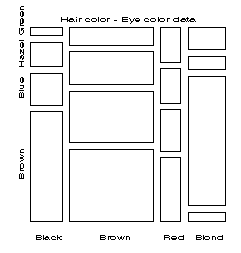Figure 5: Condensed column proportion mosaic

## Detecting patterns

In Hartigan & Kleiner's (1981) original version ( Figure 5), all the tiles are unshaded and drawn in one color, so only the relative sizes of the rectangles indicate deviations from independence. Friendly (1991b) shows how to increase the visual impact of the mosaic by using color and shading to reflect the size of the residual, and by reordering rows and columns to make the pattern more coherent. The resulting display shows both the observed frequencies and the pattern of deviations from a specified model.

### Displaying residuals

Figure 6 gives the extended the mosaic plot, showing the standardized deviation from independence, d sub ij by the color and shading of each rectangle: cells with positive deviations are drawn black, outlined with solid lines, with shading slanted from upper left to lower right (NE to SW); negative deviations are drawn red, outlined with broken lines and shaded SE-NW. The absolute value of the deviation is portrayed by shading density: cells with absolute values less than 2 are empty; cells with | d sub ij | >= 2 are filled; those with | d sub ij | >= 4 are filled with a darker pattern. Standardized deviations are often referred to a standard Gaussian distribution. Under the assumption of independence, these values roughly correspond to two-tailed probabilities p < .05 and p < .0001 that a given value of | d sub ij | exceeds 2 or 4.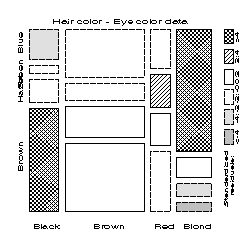Figure 6: Enhanced mosaic, reordered and shaded

### Reordering categories

When the row or column variables are unordered, we are also free to rearrange the corresponding categories in the plot to help show the nature of association. For example, in Figure 6, the eye color categories have been permuted so that the deviations from independence have an opposite-corner pattern, with positive values running from SW to NE corners, negative values along the opposite diagonal. Coupled with size and shading of the tiles, the excess in the black-brown and blond-blue cells, together with the underrepresentation of brown-haired blonds and people with black hair and blue eyes is now quite apparent. Though the table was reordered based on the d sub ij values, both dimensions in Figure 6 are ordered from dark to light, suggesting an explanation for the association.

## Multi-way tables

The condensed form of the mosaic plot generalizes readily to the display of multi-dimensional contingency tables. Imagine that each cell of the two-way table for hair and eye color is further classified by one or more additional variables--sex and level of education, for example. Then each rectangle can be subdivided horizontally to show the proportion of males and females in that cell, and each of those horizontal portions can be subdivided vertically to show the proportions of people at each educational level in the hair-eye-sex group.

## Fitting models

When three or more variables are represented in the mosaic, we can fit several different models of independence and display the residuals from that model. We treat these models as null or baseline models, which may not fit the data particularly well. The deviations of observed frequencies from expected, displayed by shading, will often suggest terms to be added to to an explanatory model that achieves a better fit.
• Complete independence: The model of complete independence asserts that all joint probabilities are products of the one-way marginal probabilities: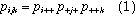for all i , j , k in a three-way table. This corresponds to the log-linear model [A] [B] [C]. Fitting this model puts all higher terms, and hence all association among the variables, into the residuals.
• Joint independence: Another possibility is to fit the model in which variable C is jointly independent of variables A and B,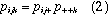This corresponds to the log-linear model [ A B ] [ C ]. Residuals from this model show the extent to which variable C is related to the combinations of variables A and B but they do not show any association between A and B.

For example, with the data from Table 1 broken down by sex, fitting the model [HairEye][Sex] allows us to see the extent to which the joint distribution of hair-color and eye-color is associated with sex. For this model, the likelihood-ratio G sup 2 is 29.35 on 15 df (p = .015), indicating some lack of fit. The three-way mosaic, shown in Figure 7, highlights two cells: males are underrepresented among people with brown hair and brown eyes, and overrepresented among people with brown hair and blue eyes. Females in these cells have the opposite patterns, with residuals just shy of +- 2. The d sub ij sup 2 for these four cells account for 15.3 of the chi sup 2 for the model [HairEye] [Sex]. Hence, except for these cells hair color and eye color appear unassociated with sex.Figure 7: Mosaic display for hair color, eye color, and sex

### Sequential plots and models

The series of mosaic plots fitting models of joint independence to the marginal subtables can be viewed as partitioning the hypothesis of complete independence in the full table.

For a three-way table, the the hypothesis of complete independence, H sub { A otimes B otimes C } can be expressed as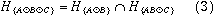where H sub { A otimes B } denotes the hypothesis that A and B are independent in the marginal subtable formed by collapsing over variable C, and H sub { AB otimes C } denotes the hypothesis of joint independence of C from the AB combinations. When expected frequencies under each hypothesis are estimated by maximum likelihood, the likelihood ratio G ²s are additive: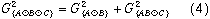For example, for the hair-eye data, the mosaic displays for the [Hair] [Eye] marginal table and the [HairEye] [Sex] table can be viewed as representing the partition
```Model                      df        G2

[Hair] [Eye]                9       146.44
[Hair, Eye] [Sex]          15        29.35
------------------------------------------
[Hair] [Eye] [Sex]         24       179.79
```

This partitioning scheme extends readily to higher-way tables.

# Correspondence analysis

Correspondence analysis is an exploratory technique related to principal components analysis that finds a multidimensional representation of the association between the row and column categories of a two-way contingency table. This technique finds scores for the row and column categories on a small number of dimensions that account for the greatest proportion of the chi sup 2 for association between the row and column categories. For graphical display, two or three dimensions are typically used to give a reduced rank approximation to the data.

For a two-way table the scores for the row categories, namely x sub im, and column categories, y sub jm, on dimension m = 1, ... , M are derived from a singular value decomposition of residuals from independence, expressed as d sub ij / sqrt n, to account for the largest proportion of the chi sup 2 in a small number of dimensions.

Thus, correspondence analysis is designed to show how the data deviate from expectation when the row and column variables are independent, as in the association plot and mosaic display. The association plot and mosaic display depict every cell in the table, however, and for large tables it may be difficult to see patterns. Correspondence analysis shows only row and column categories in the two (or three) dimensions which account for the greatest proportion of deviation from independence.

In SAS Version 6, correspondence analysis is performed using PROC CORRESP in SAS/STAT. An OUT= data set from PROC CORRESP contains the row and column coordinates, which can be plotted with PROC PLOT or PROC GPLOT. The program below reads the hair and eye color data into the data set COLORS, and calls the CORRESP procedure.

```data colors;
input BLACK BROWN RED BLOND   EYE \$;
cards;
68  119   26    7     Brown
20   84   17   94     Blue
15   54   14   10     Hazel
5   29   14   16     Green
;
proc corresp data=colors out=coord short;
var BLACK BROWN RED BLOND;
id eye;
```

The printed output from the CORRESP procedure indicates that over 98% of the chi sup 2 for association is accounted for by two dimensions, with most of that attributed to the first dimension. A plot of the row and column points, shown in Figure 8, can be constructed from the OUT= data set COORD requested in the PROC CORRESP step. The plot shows that both hair color and eye color vary from dark to light across Dimension 1, confirming the impression from the mosaic display. Dimension 2 reflects an independent association of red hair and green eyes. In fact, in the mosaic display we use scores on the first (largest) dimension to reorder the categories of variables in order to display the pattern of association most clearly.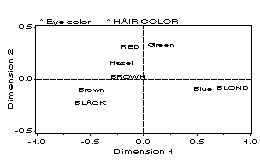Figure 8: Correspondence analysis plot

## Multi-way tables

A three- or higher-way table can be analyzed by correspondence analysis in several ways (Friendly, 1991a). One approach is called "stacking". A three-way table, of size I x J x K can be sliced into I two-way tables, each J x K. If the slices are concatenated vertically, the result is one two-way table, of size ( I x J ) x K. In effect, the first two variables are treated as a single composite variable, which represents the main effects and interaction between the original variables that were combined. Van der Heijden and de Leeuw (1985) discuss this use of correspondence analysis for multi-way tables and show how each way of slicing and stacking a contingency table corresponds to the analysis of a specified log-linear model. In particular, for the three-way table that is reshaped as a table of size ( I x J ) x K, the correspondence analysis solution analyzes residuals from the log-linear model [AB] [C].

# Effect plots for logit models

Loglinear and logit models generalize tests of association to three- and higher-way tables. A log-linear model expresses the relationship among all variables as a model for the log of the expected cell frequency. For example, for a three-way table, the hypothesis of no three-way association can be expressed as the log-linear model,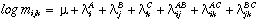The log-linear model treats the variables symmetrically: none of the variables is distinguished as a response variable. However, the association parameters may be difficult to interpret, and the absence of a dependent variable makes it awkward to plot results in terms of the log-linear model. In this case, correspondence analysis and the mosaic display provide a simpler way to display the patterns of association in a contingency table.

On the other hand, if one variable can be regarded as a response variable then the effects of the other, independent variables may be expressed as a logit model. For example, if variable C is a binary response, then the log-linear model can be expressed as an equivalent logit model,where alpha = 2 lambda sub 1 sup C, beta sub i sup A = 2 lambda sub i1 sup AC, and beta sub j sup B = 2 lambda sub j1 sup BC, because all lambda terms sum to zero.

Both log-linear and logit models can be fit using PROC CATMOD in SAS. For logit models, plots of observed and predicted logits provide an effective way to interpret a fitted model, and are easily constructed from an output data set produced by CATMOD. Fox (1987) describes general methods for constructing these plots for generalized linear models; see Friendly and Fox (1992) for further examples and comparisons of these plots with mosaic displays.

The example below analyzes the Berkeley admissions data by department to determine the source of the apparent gender bias in favor of males shown in the four-fold display ( Figure 4). The log-linear model [AdmitDept] [AdmitGender] [DeptGender] allows for effects of both Gender and Department on admission, and is equivalent to the logit modelModel (5) is fit using the statements below. The RESPONSE statement is used to produce an output data set, PREDICT, for plotting.

```data berkeley;
do dept = 'A','B','C','D','E','F';
do gender = 'Male  ', 'Female';
input freq @@;
output;
end; end; end;
cards;
512  313    89   19
353  207    17    8
120  205   202  391
138  279   131  244
53  138    94  299
22  351    24  317
;
proc catmod order=data data=berkeley;
weight freq;
response / out=predict;
model admit = dept gender / ml noiter;
```

The results of the PROC CATMOD step show a strong effect of Department, but none of Gender and a significant lack of fit.

```   MAXIMUM-LIKELIHOOD ANALYSIS-OF-VARIANCE TABLE

Source                 DF   Chi-Square      Prob
-------------------------------------------------
INTERCEPT               1       262.49    0.0000
GENDER                  1         1.53    0.2167
DEPT                    5       534.78    0.0000

LIKELIHOOD RATIO        5        20.20    0.0011
```

To interpret these results we plot the observed and predicted values for each Dept-Gender group. The response variable has a simple, additive form (5) on the logit scale (log odds), but is easier to understand on the probability scale. One compromise is to plot results on the logit scale, adding a second scale showing probability values. The data set PREDICT contains observed (_OBS_) and predicted (_PRED_) values, and estimated standard errors (_SEPRED_) on both scales. The logit values have _TYPE_ = 'FUNCTION'.

```DEPT GENDER ADMIT _TYPE_    _OBS_  _PRED_ _SEPRED_

A   Male         FUNCTION  0.492   0.582   0.069
A   Male   Admit PROB      0.621   0.642   0.016
A   Male   Rejec PROB      0.379   0.358   0.016
A   Female       FUNCTION  1.544   0.682   0.099
A   Female Admit PROB      0.824   0.664   0.022
A   Female Rejec PROB      0.176   0.336   0.022
...
```
To plot the fitted logits, select the _TYPE_ = 'FUNCTION' observations in a data step:
```data predict;
set predict;
if _type_ = 'FUNCTION';
```
A simple plot of predicted logits can then be obtained as a plot of _pred_ * dept = gender in a PROC GPLOT step. The plot displayed in Figure 9 uses the Annotate facility to add 95% confidence limits, calculated as ` _pred_ +- 1.96 _sepred_`, and a probability scale at the right. These steps are combined in a macro program, CATPLOT, used as follows:
```%catplot(data=predict, class=gender, xc=dept,
z=1.96, anno=pscale)
```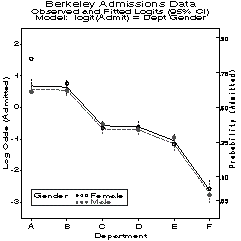Figure 9: Effects of Gender and Department on Admission

The effects shown in Figure 9 for each department contradict the apparent gender bias shown in the aggregate data; in fact, the predicted odds of admission is slightly higher for females than males. The resolution of this contradiction (an example of Simpson's paradox) can be found in the large differences in admission rates among departments. Men and women apply to different departments differentially, and in these data women apply in larger numbers to departments that have a low acceptance rate. The aggregate results are misleading because they falsely assume men and women are equally likely to apply in each field. (This explanation ignores the possibility of structural bias against women, e.g., lack of resources allocated to departments that attract women applicants.)

These effects may all be seen in Figure 10, a mosaic display of the data showing observed frequencies and residuals from the log-linear model [AdmitDept] [GenderDept] which asserts that admission and gender are conditionally independent, given department (equivalent to logit (Admit) = alpha + beta sub i sup DEPT). The four large blocks corresponding to admission by gender show the greater overall acceptance of males than females. Among admitted applicants, however, there are larger proportions of women in the departments (C-F) with low admission rates. The lack of fit of model [AD] [GD] is concentrated entirely in Department A, where a greater proportion of females is admitted.Figure 10: Mosaic display of Berkeley admissions data

### Acknowledgements

I am grateful to John Fox and Paul Herzberg for careful readings of an initial draft of this paper.

For further information, contact:
Michael Friendly Psychology Department, York University Downsview, ONT, Canada M3J 1P3
email: <friendly@YorkU.CA>
www: http://www.math.yorku.ca/SCS/friendly.html

# References

1. Bickel, P. J., Hammel, J. W. & O'Connell, J. W. (1975). Sex bias in graduate admissions: data from Berkeley. Science, 187, 398-403.
2. Cohen, A. (1980). On the graphical display of the significant components in a two-way contingency table. Commun. Statist.-Theor. Meth., A9, 1025-1041.
3. Fox, J. (1987). Effect displays for generalized linear models. In C. C. Clogg (Ed.), Sociological Methodology, 1987, 347-361. San Francisco: Jossey-Bass.
4. Friendly, M. (1991a). SAS System for Statistical Graphics. Cary, NC: SAS Institute Inc.
5. Friendly, M. (1991b), Mosaic displays for multi-way contingency tables. York Univ.: Dept. of Psychology Reports, 1991, No. 195.
6. Friendly, M. (1992), SAS macro programs for statistical graphics. Psychometrika, 313-317.
7. Friendly, M. and Fox, J. (1992). Interpreting higher order interactions in log-linear analysis: A picture is worth 1000 numbers. York Univ.: Inst. for Social Research Report.
8. Hartigan, J. A., and Kleiner, B. (1981). Mosaics for contingency tables. In W. F. Eddy (Ed.), Computer Science and Statistics: Proceedings of the 13th Symposium on the Interface. New York: Springer-Verlag.
9. Heijden, P. G. M. van der, and de Leeuw, J. (1985). Correspondence analysis used complementary to loglinear analysis. Psychometrika, 50, 429-447.
10. Riedwyl, H., & Schupbach, M. (1983). Siebdiagramme: Graphische Darstellung von Kontingenztafeln. Technical Report No. 12, Institute for Mathematical Statistics, University of Bern, Bern, Switzerland.
11. Snee, R. D. (1974). Graphical display of two-way contingency tables. The American Statistician, 28, 9-12.